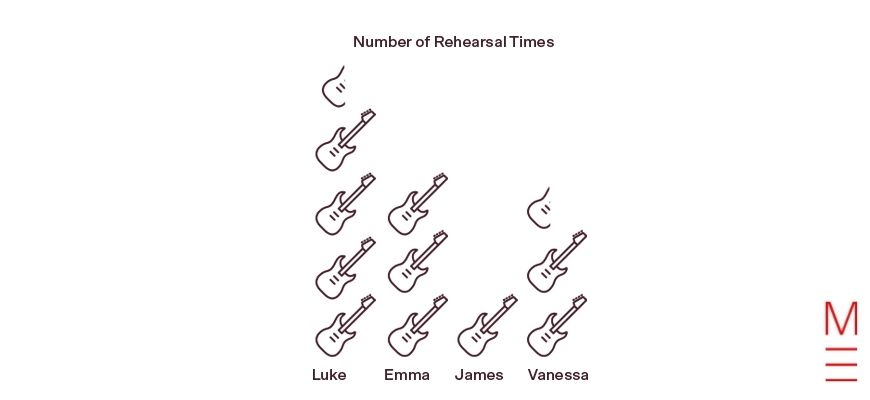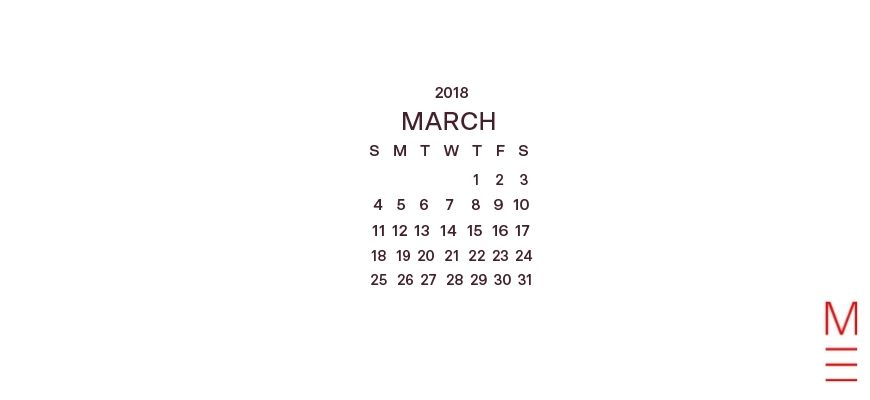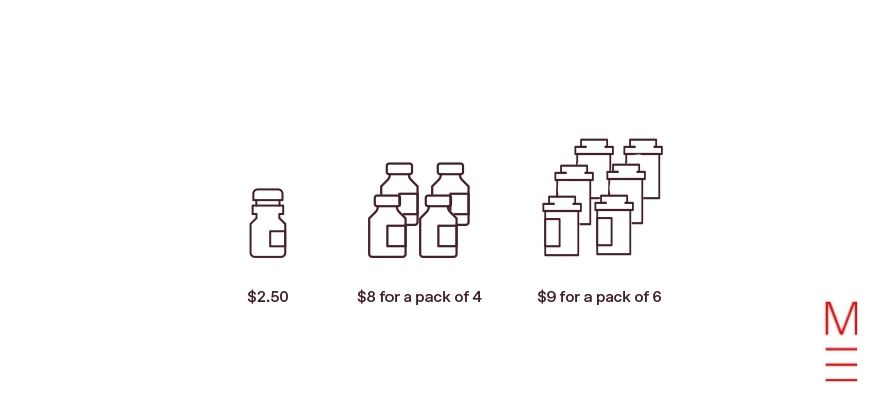# 5 Common Numeracy Mistakes Students Make in the Year 3 NAPLAN

Wondering what the Year 3 NAPLAN Numeracy test is all about? Not too sure how to prepare your child for it?In this article, we’ll discuss some of the most common numeracy mistakes students make in the Year 3 NAPLAN and how to avoid them. That way, your child can know what types of questions and pitfalls to look out for.

## What is the Year 3 NAPLAN numeracy test?

So, first off, what is the NAPLAN numeracy test?

• The NAPLAN Numeracy test is 45-minutes long.
• There are 35 questions consisting of multiple choice and constructed responses.

NAPLAN tests can be sat either in a paper or online format.

Unlike paper tests, online exams involve technology-enhanced items, delivering questions of different skill levels that cater accordingly to students’ responses and abilities. If you want to learn more about NAPLAN read our article, here.

### What does NAPLAN assess?

Well, the numeracy test aims to assess students’ problem-solving and reasoning skills, their fluency, and their ability to understand and comprehend mathematical problems.

You can expect topics such as number and algebra, measurement and geometry, as well as statistics and probability to appear in your child’s NAPLAN test.

You can find practice NAPLAN tests and a simulator on the NAPLAN website.

## 5 Common Numeracy Mistakes Students Make in the Year 3 NAPLAN

So, now that you have a general understanding of what the numeracy test consists of, let’s discuss 5 common numeracy mistakes in the Year 3 NAPLAN test.

## 1. Incorrectly interpreting graph questions

A common type of trick question in NAPLAN numeracy tests involves interpreting graphs.

### What does this mean?

In some instances, students must be able to count the number of objects or items in a graph.

Then they must perform basic addition or subtraction to calculate the total number of objects that are asked in the question.

Sounds pretty simple right?

Well, sometimes it isn’t all this straightforward.

Some questions may involve graphs with a different key or scale, which means that individual objects can represent more than one item.

Still a bit confused?

Let’s take a look at the example question below.

### Example question:

Four friends were counting the number of times they practised playing their instrument that week.

This graph shows how many times each of the friends rehearsed.Vanessa rehearsed 5 times.

Emma rehearsed 6 times.

How many more times did Luke rehearse than James?

### Solution:

Taking a look at the graph, we see that 2.5 guitars correlate to the 5 times Vanessa rehearsed.

Similarly, the 3 guitars correlate to the 6 times Emma rehearsed.

With basic multiplication, we recognise that 1 guitar = 2 times.

Therefore, to calculate how many more times Luke has rehearsed than James, you need to calculate how many times Luke and James have rehearsed individually.

Looking at the graph, Luke has 4.5 guitars. This correlates to 9 times.

On the other hand, James has 1 guitar, which correlates to 2 times.

So  $$9 \ – 2 = 7$$

As such, Luke has rehearsed 7 more times than James.

A common numeracy mistake students often make is counting the number of guitars in the graph as individual rehearsal times.

This would mean that Luke’s 4.5 guitars = 4.5 times (which is incorrect).

Therefore, your child must be wary that graphs may not always use the same scale or key, and it is important to check that numbers in the question correlate to the graph provided.

## 2. Counting days and weeks

Another common numeracy mistake often made by Year 3 students sitting the NAPLAN test is related to interpreting calendars.

To prepare for this, your child should practise learning how to read and interpret calendars.

This includes understanding the number of days in a month, days of a week, and dates.

Let’s take a look at the example below of a question that some students often struggle with.

### Example question:

Aaron’s birthday is on the 16th of March.

His sister’s birthday was twenty-two days ago.Which day of the week was his sister’s birthday in 2018?

### Solution:

Taking a look at the calendar above, if we try to count back 22 days from 16 March, Aaron’s sister’s birthday does not lie in March.

Now, many students would be stuck and unsure of where to go from here.

That is why it is important for your child to recognise that they can continue ‘counting backwards’, regardless of whether the date goes beyond the current month.

Thursday 1st of March is 15 days from Aaron’s birthday.

$$22 \ – 15 = 7$$

Therefore, the correct answer is Thursday. And so, continuing to go backwards for 7 days would mean his sister’s birthday lands on a Thursday.

## 3. Finding the best value package

Finding the cheapest or most valuable deal also comes across as one of the trickiest questions in the Year 3 NAPLAN Numeracy test.

So, what do these questions actually entail?

Essentially, students are given a set of ‘discount deal packages’, and must choose the package that provides the most value.

It can be especially confusing or difficult for your child, if they are unsure of how to approach these types of questions.

So, let’s break it down into smaller steps with this example question.

### Example question:

A shop is selling water bottles in different packs.Which package should Joe choose that gives the best value, per water bottle?

### Solution:

Often, students may be misled by the prices displayed on each package.

This may lead students to select Option 1) $2.50, since$2.50 is lower than $8 and$9.

However, your child must keep in mind that the question is asking for the best value per bottle, not the cheapest package.

So, taking a look at the package in Option 1, we see that one water bottle costs $2.50. Now, the package in Option 2 offers$8 for a pack of 4.

Performing basic division calculations, $$8 \ ÷ 4 = 2$$

Therefore, one water bottle costs $2 in Option 2. The package in Option 3 offers a deal of ‘$9 for a pack of 6’.

Now, $$9 \ ÷ 6 = 1.5$$

Therefore one water bottle in Option 3 costs $1.50. We’re trying to find the package that gives the best value. Comparing our values in Options 1, 2, and 3, we see that$1.50 is the lowest price available (per bottle).

As such, Option 3) \$9 for a pack of 6 has the best value.

## 4. Probability

Another common numeracy mistake students make in Year 3 NAPLAN is related to probability.

### What is probability?

Well, probability is the chance of a particular event occurring.

Usually, rolling a die has a $$\frac{1}{6}$$ chance of landing on a particular face.

And flipping a coin has a $$\frac{1}{2}$$ chance of landing on a heads or tails.

It is important for your child to remember these probabilities when preparing for their numeracy test.

### Example question:

Before going to a restaurant, Jerry and Eve flip a fair coin to see who gets to decide where they eat.

Eve always calls heads. She has won the last four times.

Which of the following is true for the next flip?

A) Eve is most likely to win

B) Jerry is most likely to win

C) Both are equally likely to win

### Solution:

The correct answer is C) Both are equally likely to win

Now, your child must recognise that the question has specified a ‘fair coin’ in the question.

A fair coin has a $$\frac{50}{50}$$ chance of flipping either a heads or a tails.

It is easy to be mistaken and choose A) Eve is most likely to win since she has won the last four times.

However, no matter what the previous results have been, a fair coin will always have a 50% chance of flipping heads or tails.

## 5. Complex, multi-step questions

Usually, at the end of numeracy papers, more complex, multi-step questions often appear.

These types of questions usually require students to perform multiple calculations (e.g. addition, subtraction, multiplication, division) before reaching the final answer.

Let’s take a look at the example question below to see what this means.

### Example question:

Josh and Anne picked 28 oranges together.

Anne picked 6 more oranges than Josh.

How many oranges did Anne pick?

### Solution:

At first glance, this question appears to be quite confusing since there are

Aside from the ‘guess and check’ method, your child can actually solve this question using basic arithmetic skills.

So, starting off, let’s calculate the total number of oranges both Anne and Josh would have picked if Anne did not pick 6 more oranges.

$$28 \ – 6 = 22$$ oranges

Therefore, Anne and Josh share 22 oranges.

Now, let’s divide the total number of shared oranges across Anne and Josh.

$$22 \ ÷ 2 = 11$$ oranges

Therefore, Josh has 11 oranges, and Anne has 11 oranges.

Let’s add the extra 6 oranges that Anne had picked out.

$$11 \ + 6 = 17$$ oranges

As such, Anne has picked 17 oranges.

Here is another example of a multi-step numeracy question.

### Example question:

Ellie has 5 fish tanks. Each fish tank has 12 fish in it.

She then bought one more tank.

She shared all the fish equally between the 6 tanks.

How many fish were then in each tank?

A) 7

B) 8

C) 9

D) 10

### Solution:

A common numeracy mistake students often have is counting an additional 12 fish when Ellie had bought one more tank.

This would mean there are 72 fish in total, to be divided equally across 6 tanks.

However, this is incorrect, as the question does not state that the additional tank Ellie purchased contained 12 fish.

So, to approach this question, let’s start off by calculating the total number of fish in Ellie’s fish tanks.

$$5 \times 12 = 60$$ fish

Now, the question states that she has bought one more tank.

$$5 \ + 1 = 6$$ total tanks

Now, Ellie has shared all the fish equally between the 6 tanks.

$$60 \ ÷ 6 = 10$$ fish

Therefore D) 10 is the correct answer.

If you want to learn more about how to prepare your child for the Year 3 NAPLAN, visit this article ‘7 Common Language Convention Mistakes Students Make in the Year 3 NAPLAN’.

### Get free study tips and resources delivered to your inbox.

Join 75,893 students who already have a head start.

Our website uses cookies to provide you with a better browsing experience. If you continue to use this site, you consent to our use of cookies. Read our cookies statement.